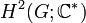# Group cohomology of groups of prime-cube order

Jump to: navigation, search

## Contents

This article gives specific information, namely, group cohomology, about a family of groups, namely: groups of prime-cube order.
View group cohomology of group families | View other specific information about groups of prime-cube order

This article describes the group cohomology of groups of prime-cube order. The two cases$p = 2$ (see group cohomology of groups of order 8) and$p = 3$ (see group cohomology of groups of order 27) are somewhat special.

## Second cohomology groups and extensions

### Schur multipliers and Schur covering groups

The Schur multiplier is defined as second cohomology group for trivial group action,$H^2(G;\mathbb{C}^\ast)$, and also as the second homology group$H_2(G;\mathbb{Z})$. A corresponding Schur covering group of$G$ is a group that arises as a stem extension with base normal subgroup the Schur multiplier and the quotient group is$G$.

Group GAP ID 2nd part Nilpotency class$H_2$ (= Schur multiplier) Order of$H_2$ Possibilities for Schur covering groups Cohomology group information Orders of Schur covering groups
cyclic group of prime-cube order 1 1 trivial group 1 cyclic group of prime-cube order --$p^3$
direct product of cyclic group of prime-square order and cyclic group of prime order 2 1 group of prime order$p$ SmallGroup(p^4,3), SmallGroup(p^4,4), SmallGroup(p^4,6) second cohomology group for trivial group action of direct product of cyclic group of prime-square order and cyclic group of prime order on cyclic group of prime order$p^4$
unitriangular matrix group:UT(3,p) 3 2 elementary abelian group of prime-square order$p^2$ lots of them second cohomology group for trivial group action of UT(3,p) on elementary abelian group of prime-square order$p^5$
semidirect product of cyclic group of prime-square order and cyclic group of prime order 4 2 group of prime order$p$ lots of them second cohomology group for trivial group action of semidirect product of cyclic group of prime-square order and cyclic group of prime order on group of prime order$p^4$
elementary abelian group of prime-cube order 5 1 elementary abelian group of prime-cube order$p^3$ lots of them second cohomology group for trivial group action of elementary abelian group of prime-cube order on elementary abelian group of prime-cube order$p^6$